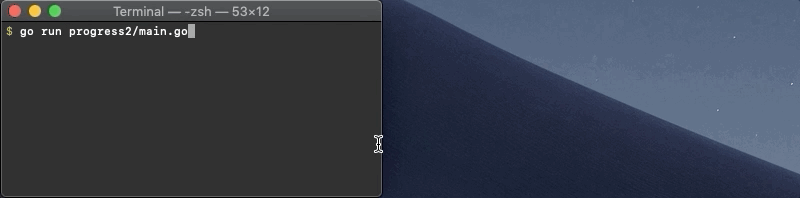## 1. 终端(terminal)的发展历史

### 1.2 VT100

VT100是一个古老的终端定义,后面出现的终端几乎都兼容这种终端.VT100无法表达颜色,因为它嵌入了单色显示器. VT100控制码是用来在终端扩展显示的代码. 比如果终端上任意坐标用不同的颜色显示字符.VT100控制码有时又称为ANSI Escape Sequence. 如果感兴趣继续了解VT的发展历史请访问vt100.net

VT100控制码ANSI Escape Sequence 顾名思义,所有控制序列开始从`\x1b` 对应逃逸上ASCII码表.今天大多数个人计算机的Telnet用户端提供最普遍的终端（一般VT100）的模拟. VT100无法表达颜色,因为它嵌入了单色显示器.但是不知道为什么VT100控制码ANSI Escape Sequence有改变颜色的控制序列的细节, 但VT241终端是高端模型嵌入彩色图形显示器.

### 1.2.1 VT100 控制码

``````\033[0m     // 关闭所有属性
\033[1m     // 设置为高亮
\033[4m     // 下划线
\033[5m     // 闪烁
\033[7m     // 反显
\033[8m     // 消隐
\033[nA     // 光标上移 n 行
\033[nB     // 光标下移 n 行
\033[nC     // 光标右移 n 行
\033[nD     // 光标左移 n 行
\033[y;xH   // 设置光标位置
\033[2J     // 清屏
\033[K      // 清除从光标到行尾的内容
\033[s      // 保存光标位置
\033[u      // 恢复光标位置
\033[?25l   // 隐藏光标
\033[?25h   // 显示光标``````

### 1.2.2 `\033[30m – \033[37m`为设置前景色

``````30: 黑色
31: 红色
32: 绿色
33: 黄色
34: 蓝色
35: 紫色
36: 青色
37: 白色``````

### 1.2.3 `\033[40m – \033[47m` 为设置背景色

``````40: 黑色
41: 红色
42: 绿色
43: 黄色
44: 蓝色
45: 紫色
46: 青色
47: 白色``````

ANSI / VT100控制码文档

## 2. Go语言终端colorful-text

``````package main
import "fmt"
func main() {
fmt.Print("\x1b[4;30;46m")//设置颜色样式
fmt.Print("Hello World")//打印文本内容
fmt.Println("\x1b[0m")//样式结束符,清楚之前的显示属性
}
``````

## 3. Go语言终端进度条progress

``````package main

import (
"fmt"
"strings"
"time"
)

func renderbar(count, total int) {
barwidth := 30
done := int(float64(barwidth) * float64(count) / float64(total))

fmt.Printf("Progress: \x1b[33m%3d%%\x1b[0m ", count*100/total)
fmt.Printf("[%s%s]",
strings.Repeat("=", done),
strings.Repeat("-", barwidth-done))
}

func main() {
total := 50
for i := 1; i <= total; i++ {
//<ESC>表示ASCII“转义”字符，0x1B
fmt.Print("\x1b7")   // 保存光标位置 保存光标和Attrs <ESC> 7
fmt.Print("\x1b[2k") // 清空当前行的内容 擦除线<ESC> [2K
renderbar(i, total)
time.Sleep(50 * time.Millisecond)
fmt.Print("\x1b8") // 恢复光标位置 恢复光标和Attrs <ESC> 8
}
fmt.Println()
}
``````

## 4. 关于终端仿真器的窗口大小

``````type winsize struct {
Row uint16
Col uint16
X  uint16
Y uint16
}

func getWinSize(fd int) (row, col uint16, err error) {
var ws *winsize
retCode, _, errno := syscall.Syscall(
syscall.SYS_IOCTL, uintptr(fd),
uintptr(syscall.TIOCGWINSZ),
uintptr(unsafe.Pointer(ws)))
if int(retCode) == -1 {
panic(errno)
}
return ws.Row, ws.Col, nil
}
``````

``````package main

import (
"fmt"
"strings"
"syscall"
"time"

"golang.org/x/sys/unix"
)

var wscol = 30

func init() {
ws, err := unix.IoctlGetWinsize(syscall.Stdout, unix.TIOCGWINSZ)
if err != nil {
panic(err)
}
wscol = int(ws.Col)
}

func renderbar(count, total int) {
barwidth := wscol - len("Progress: 100% []")
done := int(float64(barwidth) * float64(count) / float64(total))

fmt.Printf("Progress: \x1b[33m%3d%%\x1b[0m ", count*100/total)
fmt.Printf("[%s%s]",
strings.Repeat("=", done),
strings.Repeat("-", barwidth-done))
}

func main() {
total := 50
for i := 1; i <= total; i++ {
fmt.Print("\x1b7")   // save the cursor position
fmt.Print("\x1b[2k") // erase the current line
renderbar(i, total)
time.Sleep(50 * time.Millisecond)
fmt.Print("\x1b8") // restore the cursor position
}
fmt.Println()
}
``````

``````package main

import (
"fmt"
"os"
"os/signal"
"strings"
"syscall"
"time"

"golang.org/x/sys/unix"
)

var (
total = 50
count = 0
wscol = 20
)

func init() {
err := updateWSCol()
if err != nil {
panic(err)
}
}

func updateWSCol() error {
ws, err := unix.IoctlGetWinsize(syscall.Stdout, unix.TIOCGWINSZ)
if err != nil {
return err
}
wscol = int(ws.Col)
return nil
}

func renderbar() {
fmt.Print("\x1b7")       // 保存光标位置
fmt.Print("\x1b[2k")     // 清除当前行内容
defer fmt.Print("\x1b8") // 恢复光标位置

barwidth := wscol - len("Progress: 100% []")
done := int(float64(barwidth) * float64(count) / float64(total))

fmt.Printf("Progress: \x1b[33m%3d%%\x1b[0m ", count*100/total)
fmt.Printf("[%s%s]",
strings.Repeat("=", done),
strings.Repeat("-", barwidth-done))
}

func main() {
// set signal handler
sigwinch := make(chan os.Signal, 1)
defer close(sigwinch)
signal.Notify(sigwinch, syscall.SIGWINCH)
go func() {
for {
if _, ok := <-sigwinch; !ok {
return
}
_ = updateWSCol()
renderbar()
}
}()

for count = 1; count <= 50; count++ {
renderbar()
time.Sleep(time.Second)
}
fmt.Println()
}
``````

## 结束语

tech.mojotv.cntech.mojotv.cn